# NCERT Exemplar Solutions for Class 6 Maths Chapter 7 Algebra

NCERT Exemplar Solutions for Class 6 Maths Chapter 7 Algebra are provided here. By studying the questions of NCERT Exemplar Solutions, students can enhance their execution and operate on their strengths and weaknesses. It makes the students feel confident while answering the question paper. All the answers mentioned in the NCERT Exemplar Solutions for Class 6 Maths are valid and modernised, according to the newest syllabus.

Chapter 7 – Algebra is a branch of Mathematics that substitutes letters for numbers. Algebra is about finding the unknown or putting real – life variables into equations and then solving them. In this Chapter, students are going to learn some essential topics, such as variables, equations and solutions. Equations are defined as an expression with a variable, constants and the sign of equality (=).

## Download the PDF of NCERT Exemplar Solutions for Class 6 Maths Chapter 7 Algebra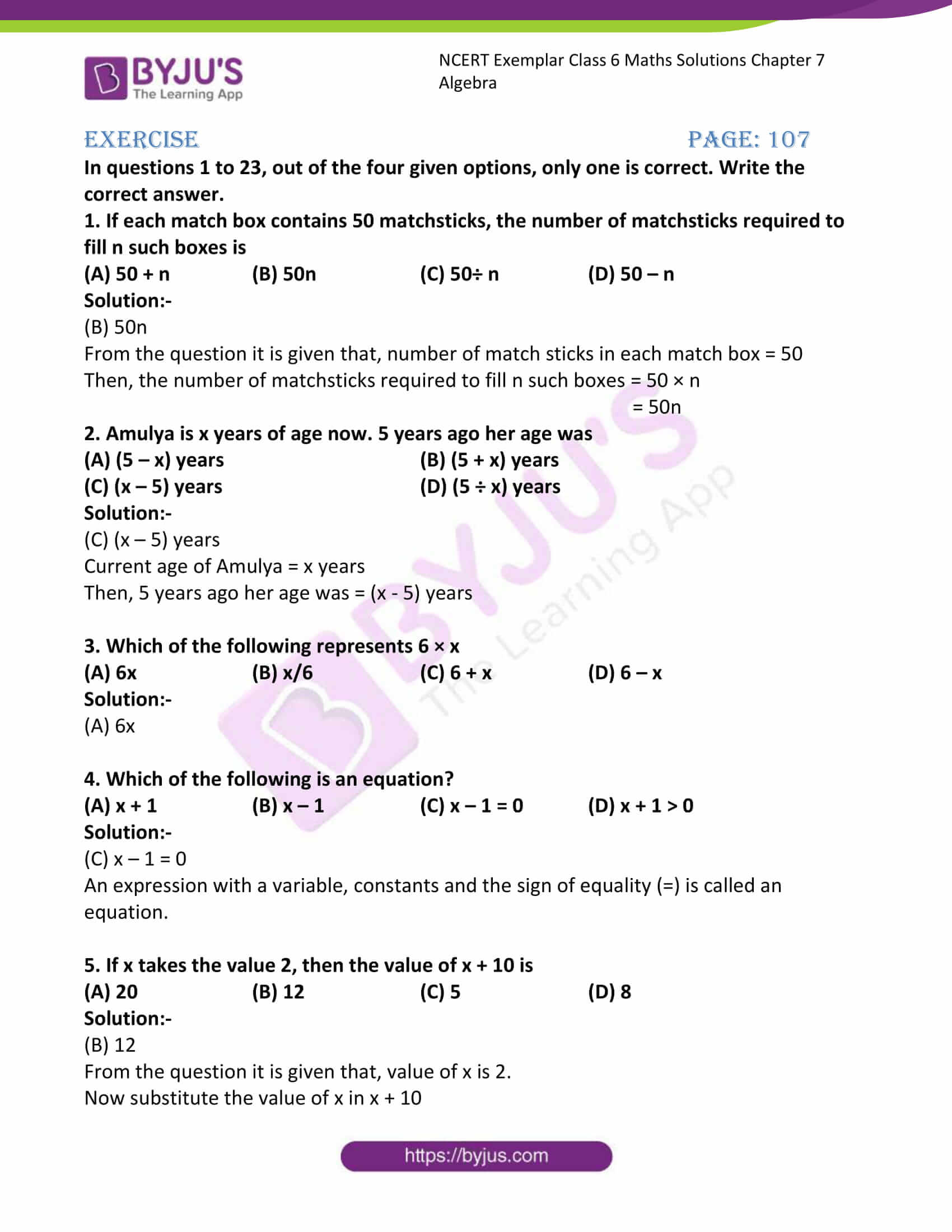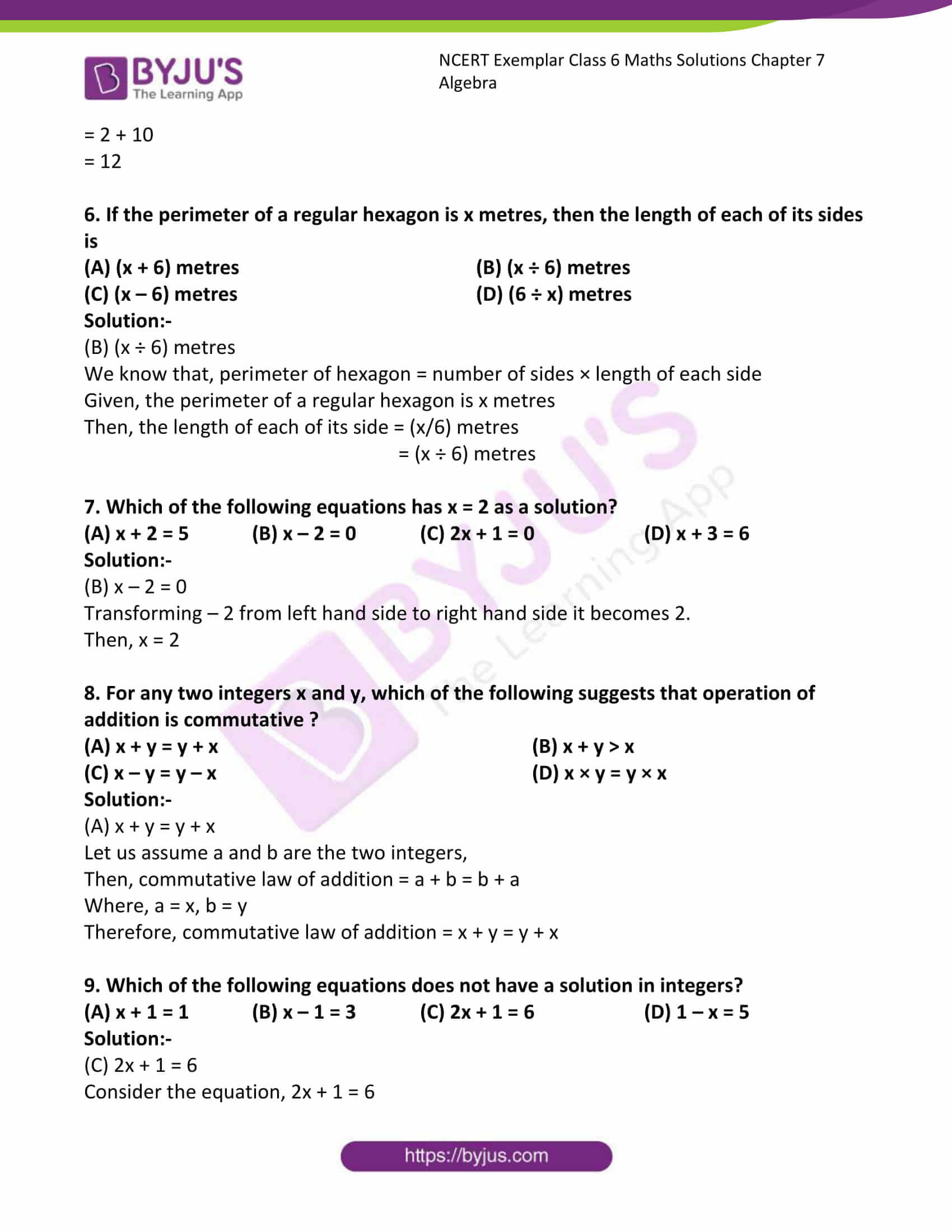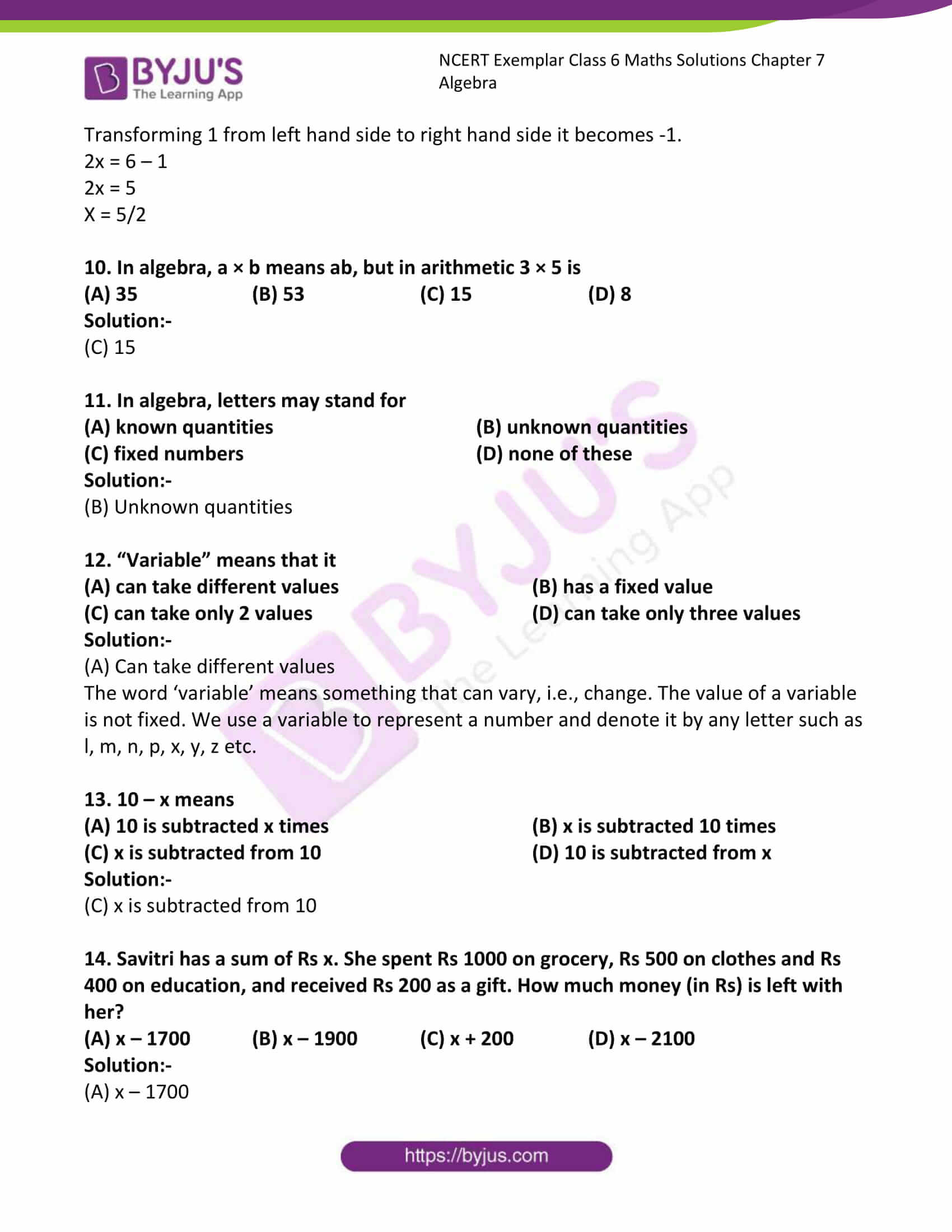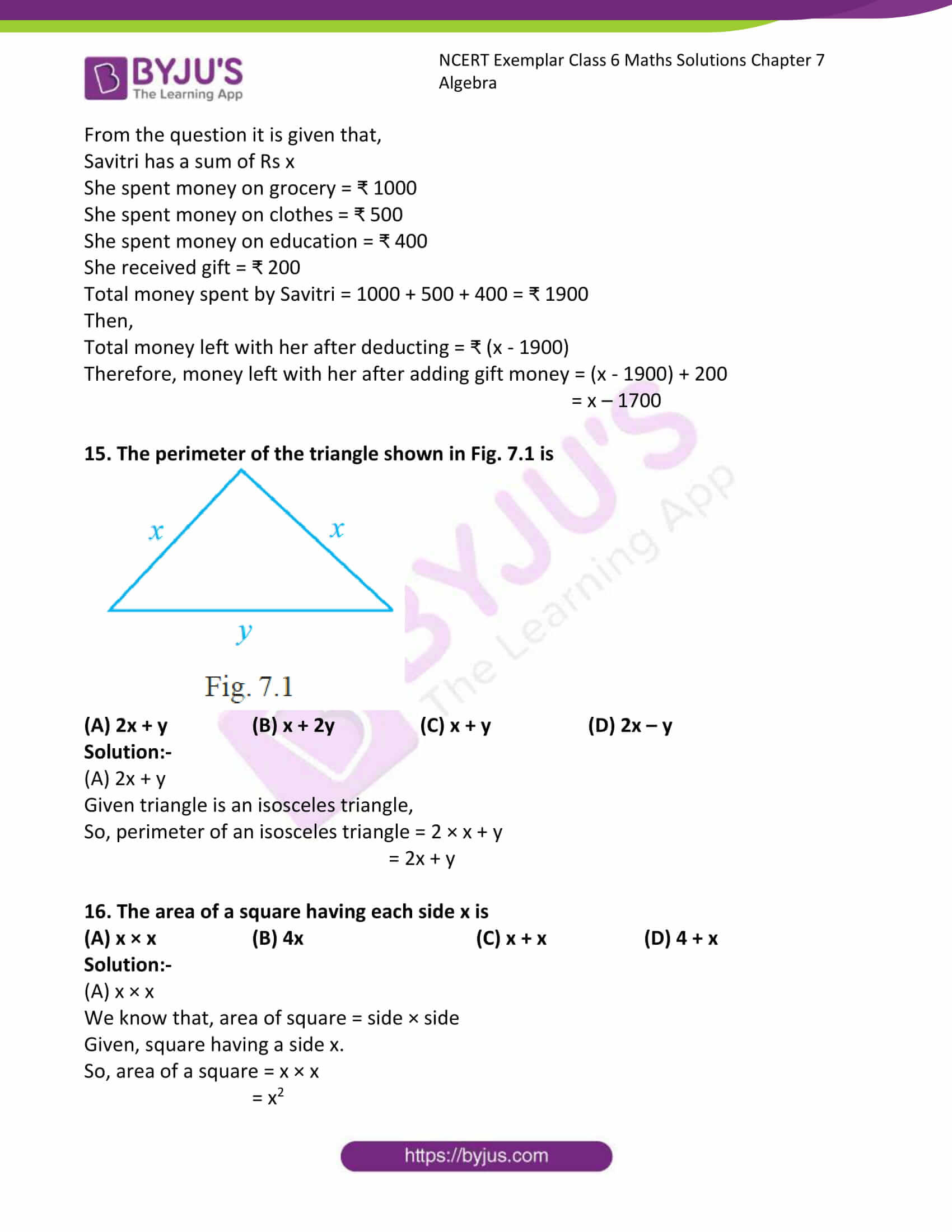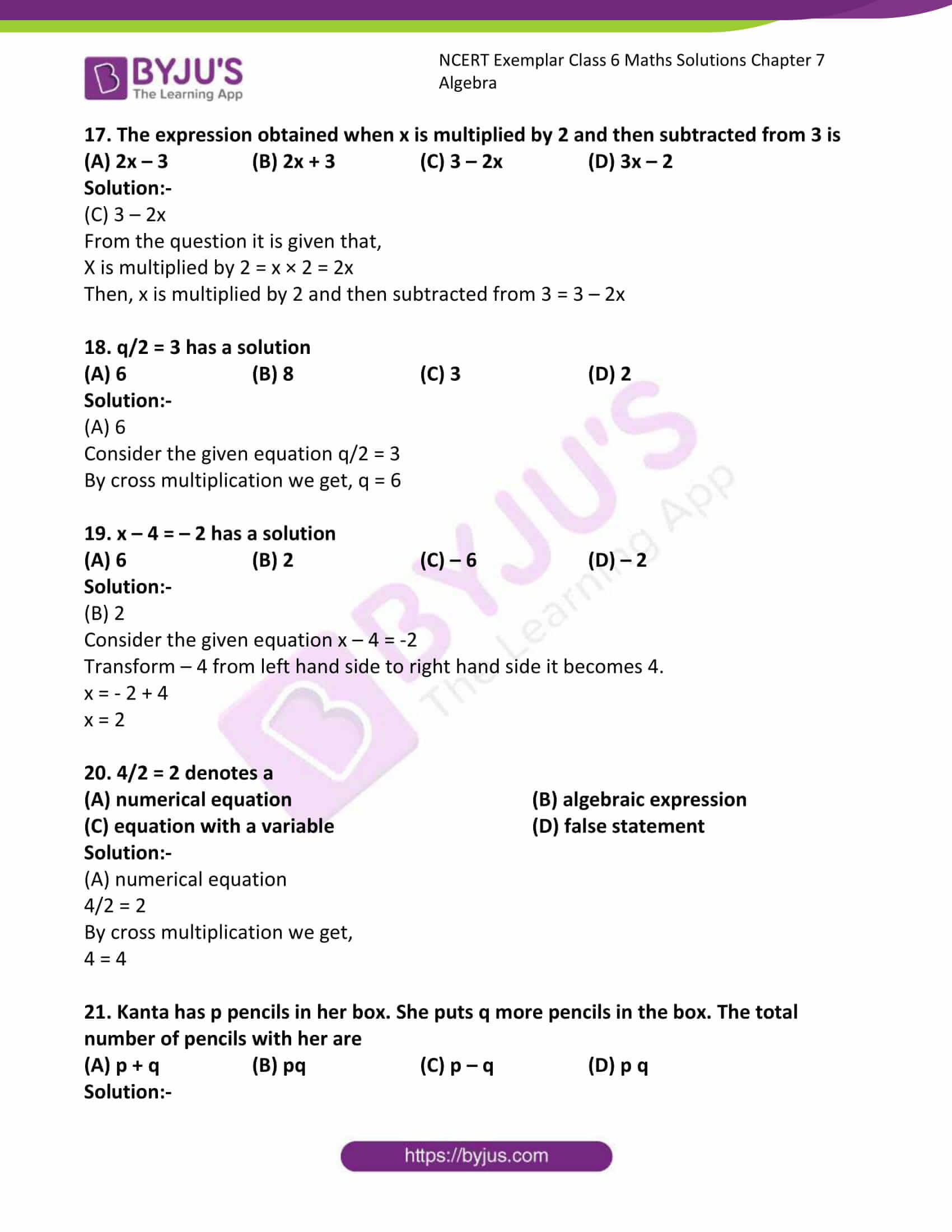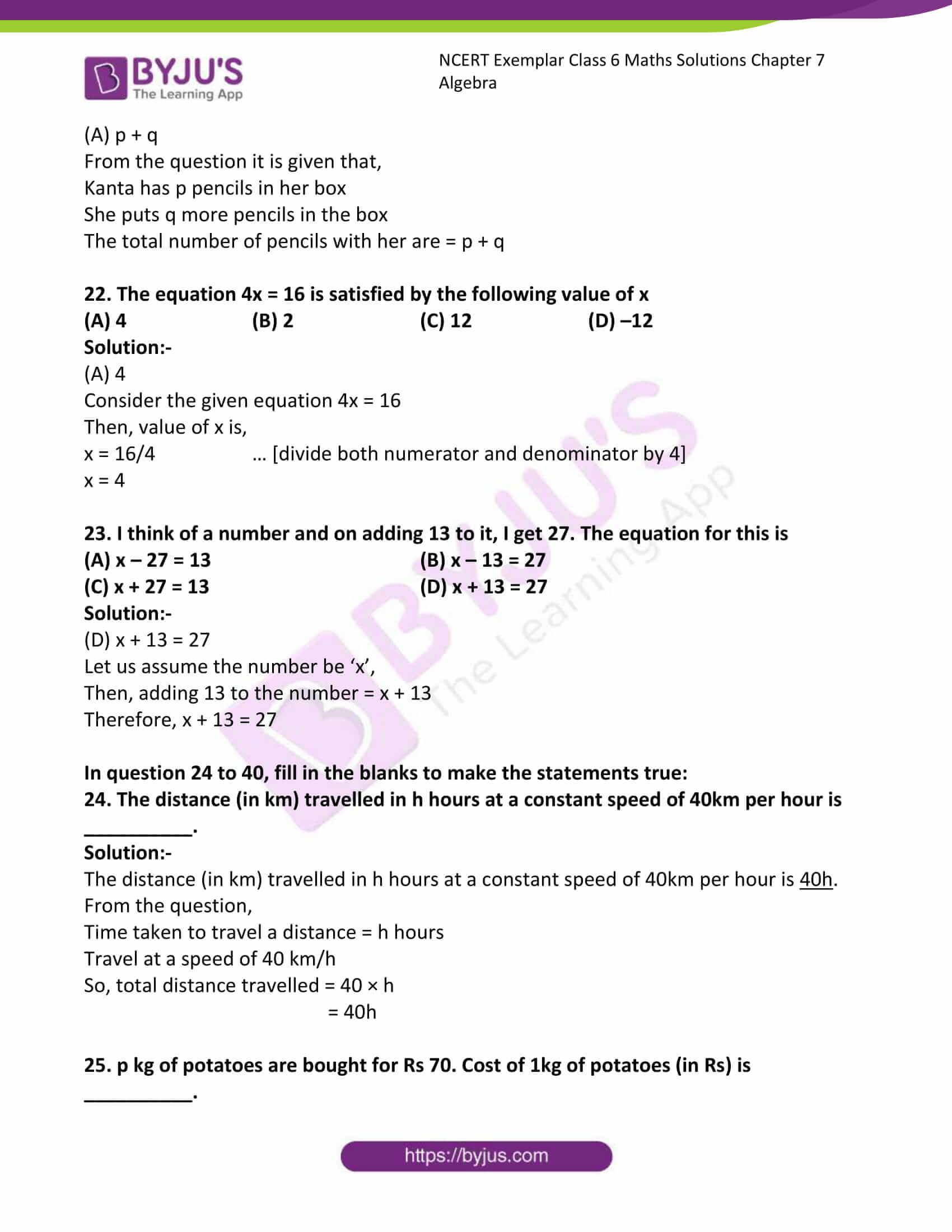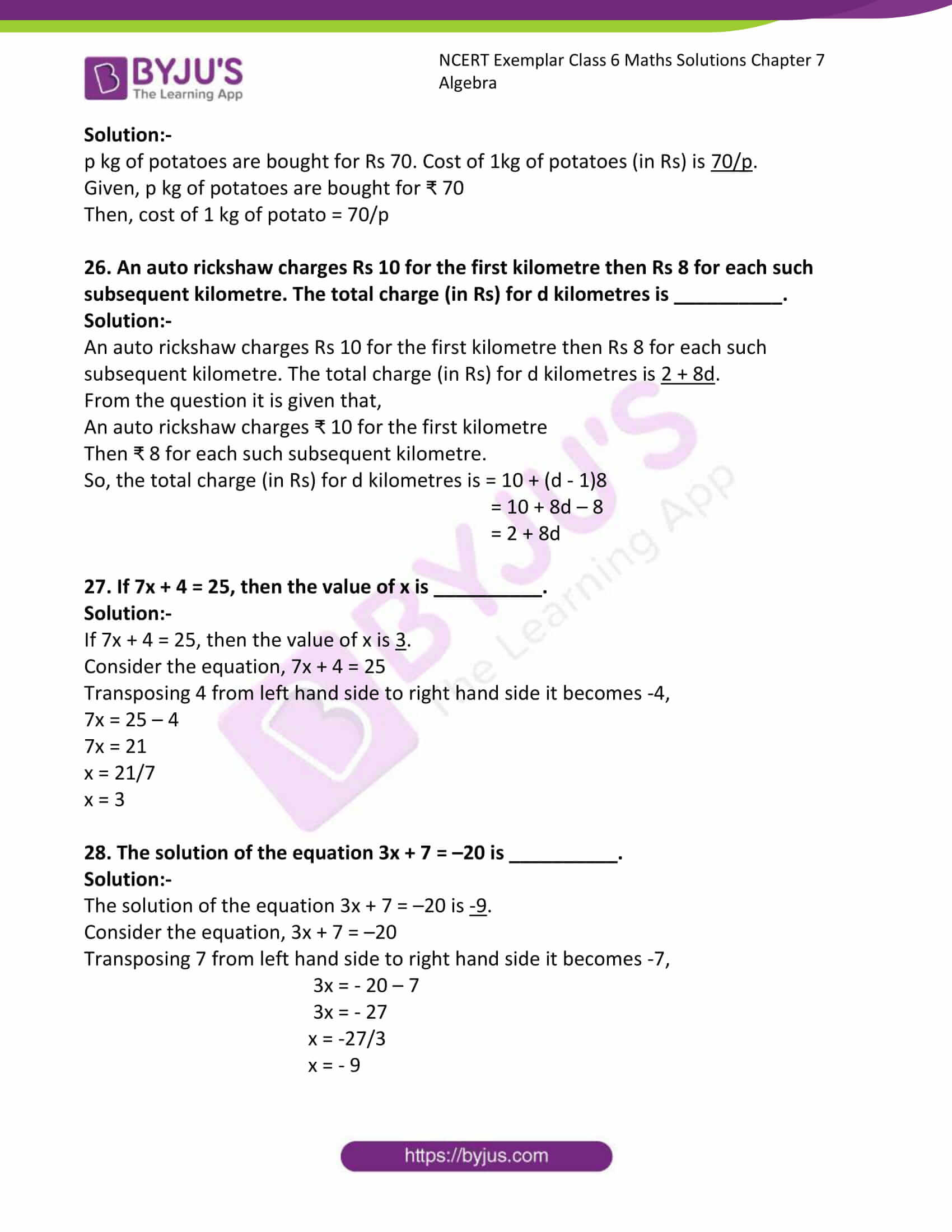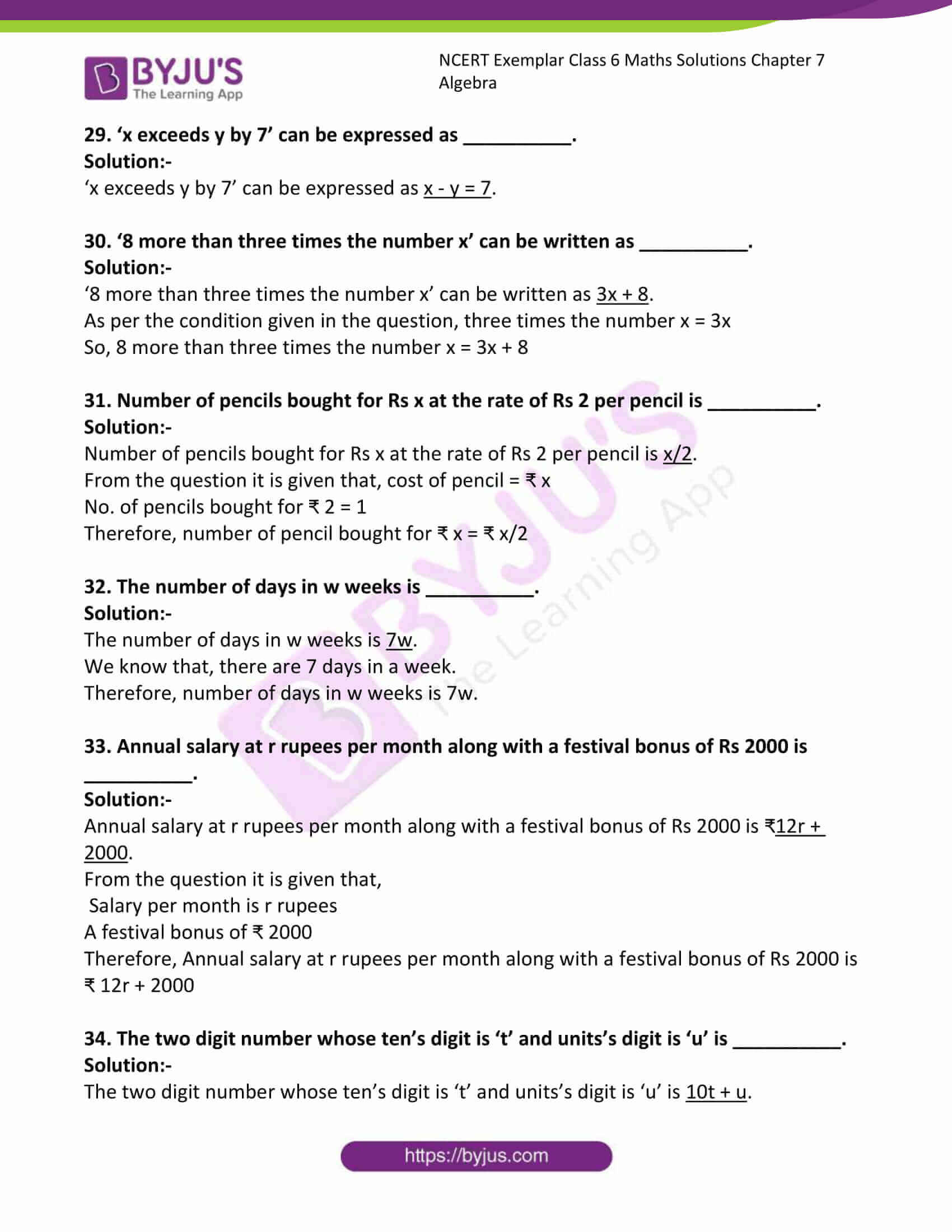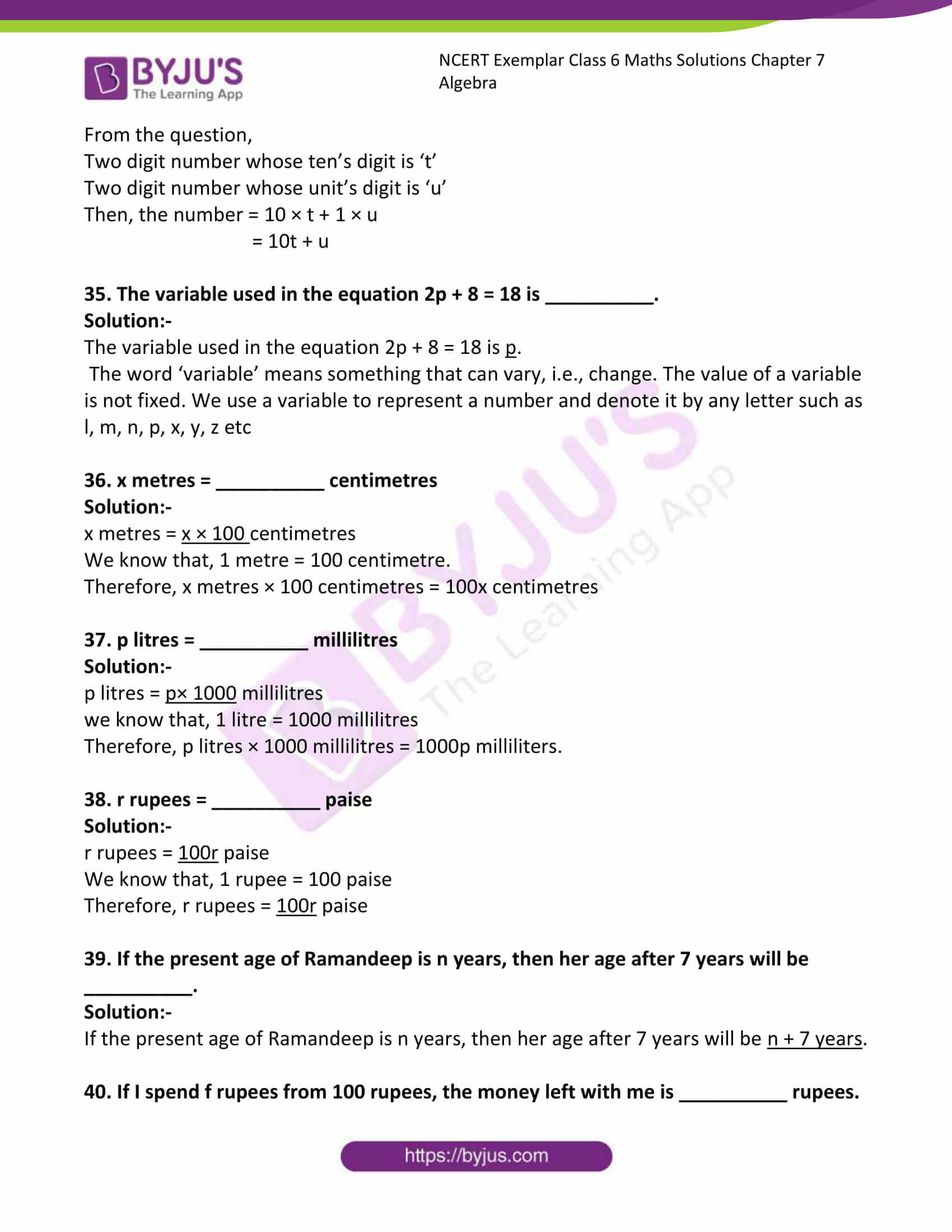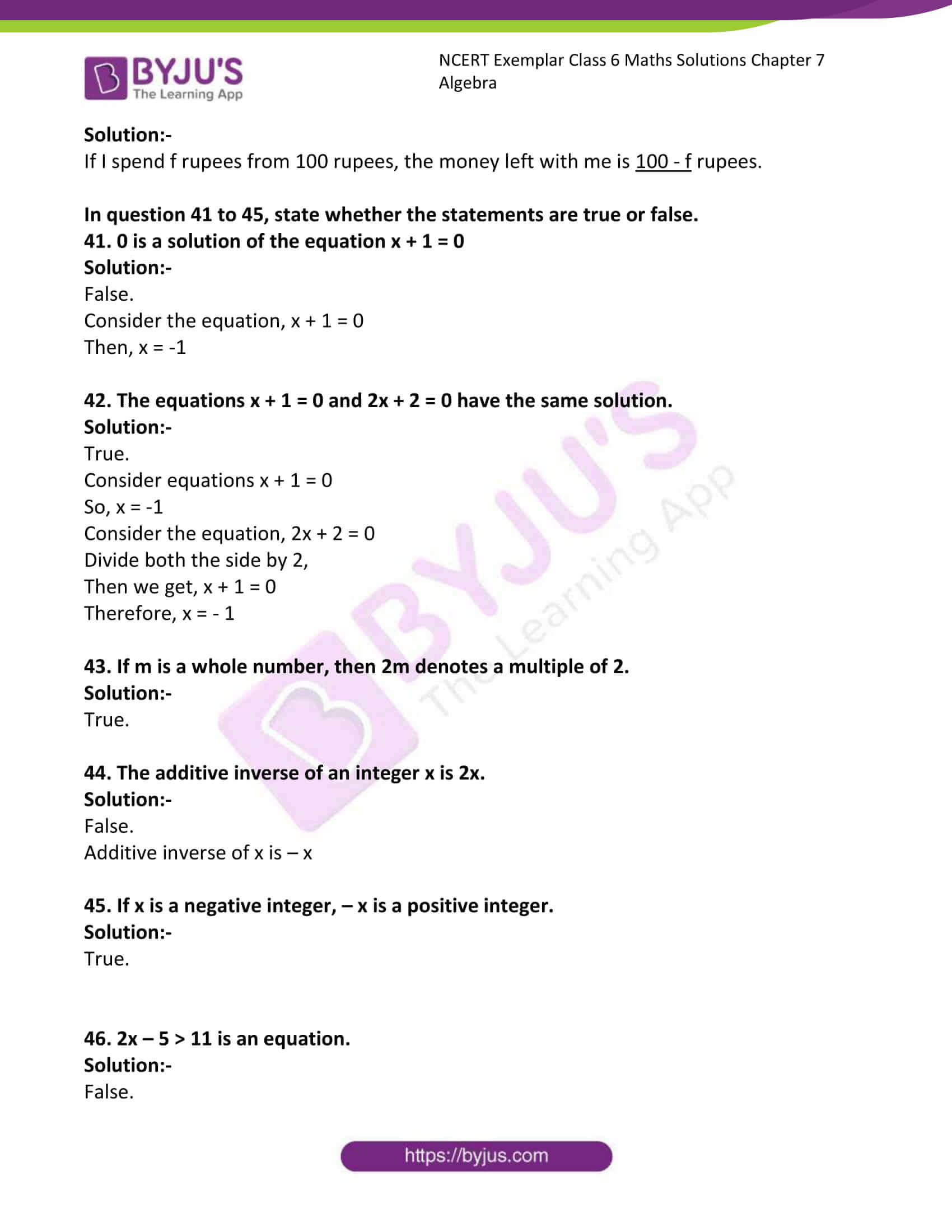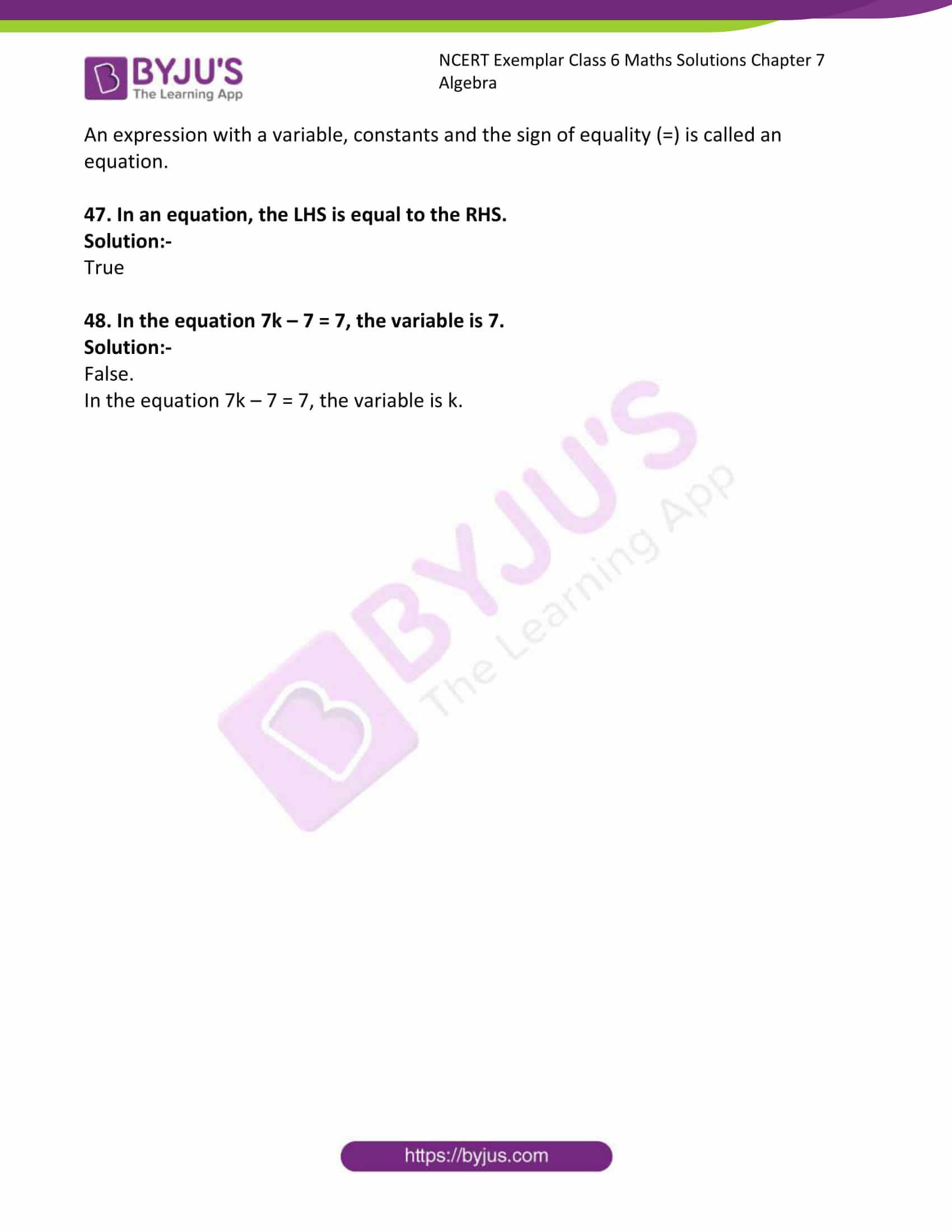### Access answers to Maths NCERT Exemplar Solutions for Class 6 Maths Chapter 7 Algebra

Exercise Page: 107

In questions 1 to 23, out of the four given options, only one is correct. Write the correct answer.

1. If each match box contains 50 matchsticks, the number of matchsticks required to fill n such boxes is

(A) 50 + n (B) 50n (C) 50÷ n (D) 50 – n

Solution:-

(B) 50n

From the question it is given that, number of match sticks in each match box = 50

Then, the number of matchsticks required to fill n such boxes = 50 × n

= 50n

2. Amulya is x years of age now. 5 years ago her age was

(A) (5 – x) years (B) (5 + x) years

(C) (x – 5) years (D) (5 ÷ x) years

Solution:-

(C) (x – 5) years

Current age of Amulya = x years

Then, 5 years ago her age was = (x – 5) years

3. Which of the following represents 6 × x

(A) 6x (B) x/6 (C) 6 + x (D) 6 – x

Solution:-

(A) 6x

4. Which of the following is an equation?

(A) x + 1 (B) x – 1 (C) x – 1 = 0 (D) x + 1 > 0

Solution:-

(C) x – 1 = 0

An expression with a variable, constants and the sign of equality (=) is called an equation.

5. If x takes the value 2, then the value of x + 10 is

(A) 20 (B) 12 (C) 5 (D) 8

Solution:-

(B) 12

From the question it is given that, value of x is 2.

Now substitute the value of x in x + 10

= 2 + 10

= 12

6. If the perimeter of a regular hexagon is x metres, then the length of each of its sides is

(A) (x + 6) metres (B) (x ÷ 6) metres

(C) (x – 6) metres (D) (6 ÷ x) metres

Solution:-

(B) (x ÷ 6) metres

We know that, perimeter of hexagon = number of sides × length of each side

Given, the perimeter of a regular hexagon is x metres

Then, the length of each of its side = (x/6) metres

= (x ÷ 6) metres

7. Which of the following equations has x = 2 as a solution?

(A) x + 2 = 5 (B) x – 2 = 0 (C) 2x + 1 = 0 (D) x + 3 = 6

Solution:-

(B) x – 2 = 0

Transforming – 2 from left hand side to right hand side it becomes 2.

Then, x = 2

8. For any two integers x and y, which of the following suggests that operation of addition is commutative ?

(A) x + y = y + x (B) x + y > x

(C) x – y = y – x (D) x × y = y × x

Solution:-

(A) x + y = y + x

Let us assume a and b are the two integers,

Then, commutative law of addition = a + b = b + a

Where, a = x, b = y

Therefore, commutative law of addition = x + y = y + x

9. Which of the following equations does not have a solution in integers?

(A) x + 1 = 1 (B) x – 1 = 3 (C) 2x + 1 = 6 (D) 1 – x = 5

Solution:-

(C) 2x + 1 = 6

Consider the equation, 2x + 1 = 6

Transforming 1 from left hand side to right hand side it becomes -1.

2x = 6 – 1

2x = 5

X = 5/2

10. In algebra, a × b means ab, but in arithmetic 3 × 5 is

(A) 35 (B) 53 (C) 15 (D) 8

Solution:-

(C) 15

11. In algebra, letters may stand for

(A) known quantities (B) unknown quantities

(C) fixed numbers (D) none of these

Solution:-

(B) Unknown quantities

12. “Variable” means that it

(A) can take different values (B) has a fixed value

(C) can take only 2 values (D) can take only three values

Solution:-

(A) Can take different values

The word ‘variable’ means something that can vary, i.e., change. The value of a variable is not fixed. We use a variable to represent a number and denote it by any letter such as l, m, n, p, x, y, z etc.

13. 10 – x means

(A) 10 is subtracted x times (B) x is subtracted 10 times

(C) x is subtracted from 10 (D) 10 is subtracted from x

Solution:-

(C) x is subtracted from 10

14. Savitri has a sum of Rs x. She spent Rs 1000 on grocery, Rs 500 on clothes and Rs 400 on education, and received Rs 200 as a gift. How much money (in Rs) is left with her?

(A) x – 1700 (B) x – 1900 (C) x + 200 (D) x – 2100

Solution:-

(A) x – 1700

From the question it is given that,

Savitri has a sum of Rs x

She spent money on grocery = ₹ 1000

She spent money on clothes = ₹ 500

She spent money on education = ₹ 400

Total money spent by Savitri = 1000 + 500 + 400 = ₹ 1900

Then,

Total money left with her after deducting = ₹ (x – 1900)

Therefore, money left with her after adding gift money = (x – 1900) + 200

= x – 1700

15. The perimeter of the triangle shown in Fig. 7.1 is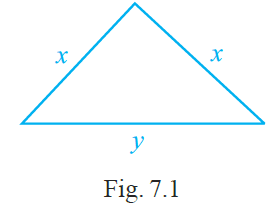(A) 2x + y (B) x + 2y (C) x + y (D) 2x – y

Solution:-

(A) 2x + y

Given triangle is an isosceles triangle,

So, perimeter of an isosceles triangle = 2 × x + y

= 2x + y

16. The area of a square having each side x is

(A) x × x (B) 4x (C) x + x (D) 4 + x

Solution:-

(A) x × x

We know that, area of square = side × side

Given, square having a side x.

So, area of a square = x × x

= x2

17. The expression obtained when x is multiplied by 2 and then subtracted from 3 is

(A) 2x – 3 (B) 2x + 3 (C) 3 – 2x (D) 3x – 2

Solution:-

(C) 3 – 2x

From the question it is given that,

X is multiplied by 2 = x × 2 = 2x

Then, x is multiplied by 2 and then subtracted from 3 = 3 – 2x

18. q/2 = 3 has a solution

(A) 6 (B) 8 (C) 3 (D) 2

Solution:-

(A) 6

Consider the given equation q/2 = 3

By cross multiplication we get, q = 6

19. x – 4 = – 2 has a solution

(A) 6 (B) 2 (C) – 6 (D) – 2

Solution:-

(B) 2

Consider the given equation x – 4 = -2

Transform – 4 from left hand side to right hand side it becomes 4.

x = – 2 + 4

x = 2

20. 4/2 = 2 denotes a

(A) numerical equation (B) algebraic expression

(C) equation with a variable (D) false statement

Solution:-

(A) numerical equation

4/2 = 2

By cross multiplication we get,

4 = 4

21. Kanta has p pencils in her box. She puts q more pencils in the box. The total number of pencils with her are

(A) p + q (B) pq (C) p – q (D) p q

Solution:-

(A) p + q

From the question it is given that,

Kanta has p pencils in her box

She puts q more pencils in the box

The total number of pencils with her are = p + q

22. The equation 4x = 16 is satisfied by the following value of x

(A) 4 (B) 2 (C) 12 (D) –12

Solution:-

(A) 4

Consider the given equation 4x = 16

Then, value of x is,

x = 16/4 … [divide both numerator and denominator by 4]

x = 4

23. I think of a number and on adding 13 to it, I get 27. The equation for this is

(A) x – 27 = 13 (B) x – 13 = 27

(C) x + 27 = 13 (D) x + 13 = 27

Solution:-

(D) x + 13 = 27

Let us assume the number be ‘x’,

Then, adding 13 to the number = x + 13

Therefore, x + 13 = 27

In question 24 to 40, fill in the blanks to make the statements true:

24. The distance (in km) travelled in h hours at a constant speed of 40km per hour is __________.

Solution:-

The distance (in km) travelled in h hours at a constant speed of 40km per hour is 40h.

From the question,

Time taken to travel a distance = h hours

Travel at a speed of 40 km/h

So, total distance travelled = 40 × h

= 40h

25. p kg of potatoes are bought for Rs 70. Cost of 1kg of potatoes (in Rs) is __________.

Solution:-

p kg of potatoes are bought for Rs 70. Cost of 1kg of potatoes (in Rs) is 70/p.

Given, p kg of potatoes are bought for ₹ 70

Then, cost of 1 kg of potato = 70/p

26. An auto rickshaw charges Rs 10 for the first kilometre then Rs 8 for each such subsequent kilometre. The total charge (in Rs) for d kilometres is __________.

Solution:-

An auto rickshaw charges Rs 10 for the first kilometre then Rs 8 for each such subsequent kilometre. The total charge (in Rs) for d kilometres is 2 + 8d.

From the question it is given that,

An auto rickshaw charges ₹ 10 for the first kilometre

Then ₹ 8 for each such subsequent kilometre.

So, the total charge (in Rs) for d kilometres is = 10 + (d – 1)8

= 10 + 8d – 8

= 2 + 8d

27. If 7x + 4 = 25, then the value of x is __________.

Solution:-

If 7x + 4 = 25, then the value of x is 3.

Consider the equation, 7x + 4 = 25

Transposing 4 from left hand side to right hand side it becomes -4,

7x = 25 – 4

7x = 21

x = 21/7

x = 3

28. The solution of the equation 3x + 7 = –20 is __________.

Solution:-

The solution of the equation 3x + 7 = –20 is -9.

Consider the equation, 3x + 7 = –20

Transposing 7 from left hand side to right hand side it becomes -7,

3x = – 20 – 7

3x = – 27

x = -27/3

x = – 9

29. ‘x exceeds y by 7’ can be expressed as __________.

Solution:-

‘x exceeds y by 7’ can be expressed as x – y = 7.

30. ‘8 more than three times the number x’ can be written as __________.

Solution:-

‘8 more than three times the number x’ can be written as 3x + 8.

As per the condition given in the question, three times the number x = 3x

So, 8 more than three times the number x = 3x + 8

31. Number of pencils bought for Rs x at the rate of Rs 2 per pencil is __________.

Solution:-

Number of pencils bought for Rs x at the rate of Rs 2 per pencil is x/2.

From the question it is given that, cost of pencil = ₹ x

No. of pencils bought for ₹ 2 = 1

Therefore, number of pencil bought for ₹ x = ₹ x/2

32. The number of days in w weeks is __________.

Solution:-

The number of days in w weeks is 7w.

We know that, there are 7 days in a week.

Therefore, number of days in w weeks is 7w.

33. Annual salary at r rupees per month along with a festival bonus of Rs 2000 is __________.

Solution:-

Annual salary at r rupees per month along with a festival bonus of Rs 2000 is ₹12r + 2000.

From the question it is given that,

Salary per month is r rupees

A festival bonus of ₹ 2000

Therefore, Annual salary at r rupees per month along with a festival bonus of Rs 2000 is ₹ 12r + 2000

34. The two digit number whose ten’s digit is ‘t’ and units’s digit is ‘u’ is __________.

Solution:-

The two digit number whose ten’s digit is ‘t’ and units’s digit is ‘u’ is 10t + u.

From the question,

Two digit number whose ten’s digit is ‘t’

Two digit number whose unit’s digit is ‘u’

Then, the number = 10 × t + 1 × u

= 10t + u

35. The variable used in the equation 2p + 8 = 18 is __________.

Solution:-

The variable used in the equation 2p + 8 = 18 is p.

The word ‘variable’ means something that can vary, i.e., change. The value of a variable is not fixed. We use a variable to represent a number and denote it by any letter such as l, m, n, p, x, y, z etc

36. x metres = __________ centimetres

Solution:-

x metres = x × 100 centimetres

We know that, 1 metre = 100 centimetre.

Therefore, x metres × 100 centimetres = 100x centimetres

37. p litres = __________ millilitres

Solution:-

p litres = p× 1000 millilitres

we know that, 1 litre = 1000 millilitres

Therefore, p litres × 1000 millilitres = 1000p milliliters.

38. r rupees = __________ paise

Solution:-

r rupees = 100r paise

We know that, 1 rupee = 100 paise

Therefore, r rupees = 100r paise

39. If the present age of Ramandeep is n years, then her age after 7 years will be __________.

Solution:-

If the present age of Ramandeep is n years, then her age after 7 years will be n + 7 years.

40. If I spend f rupees from 100 rupees, the money left with me is __________ rupees.

Solution:-

If I spend f rupees from 100 rupees, the money left with me is 100 – f rupees.

In question 41 to 45, state whether the statements are true or false.

41. 0 is a solution of the equation x + 1 = 0

Solution:-

False.

Consider the equation, x + 1 = 0

Then, x = -1

42. The equations x + 1 = 0 and 2x + 2 = 0 have the same solution.

Solution:-

True.

Consider equations x + 1 = 0

So, x = -1

Consider the equation, 2x + 2 = 0

Divide both the side by 2,

Then we get, x + 1 = 0

Therefore, x = – 1

43. If m is a whole number, then 2m denotes a multiple of 2.

Solution:-

True.

44. The additive inverse of an integer x is 2x.

Solution:-

False.

Additive inverse of x is – x

45. If x is a negative integer, – x is a positive integer.

Solution:-

True.

46. 2x – 5 > 11 is an equation.

Solution:-

False.

An expression with a variable, constants and the sign of equality (=) is called an equation.

47. In an equation, the LHS is equal to the RHS.

Solution:-

True

48. In the equation 7k – 7 = 7, the variable is 7.

Solution:-

False.

In the equation 7k – 7 = 7, the variable is k.